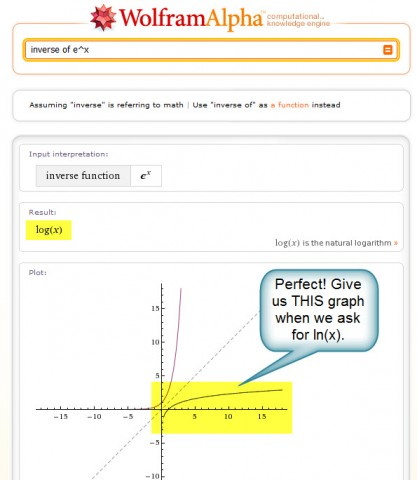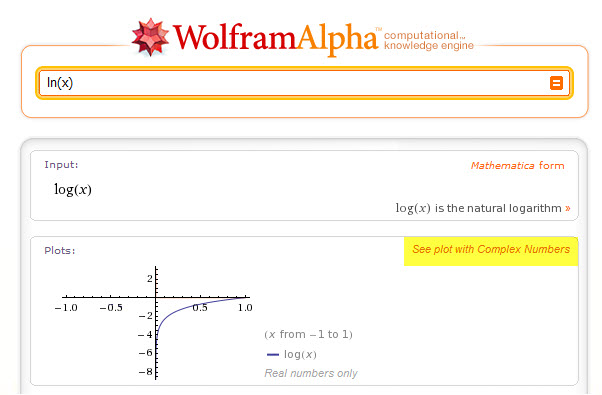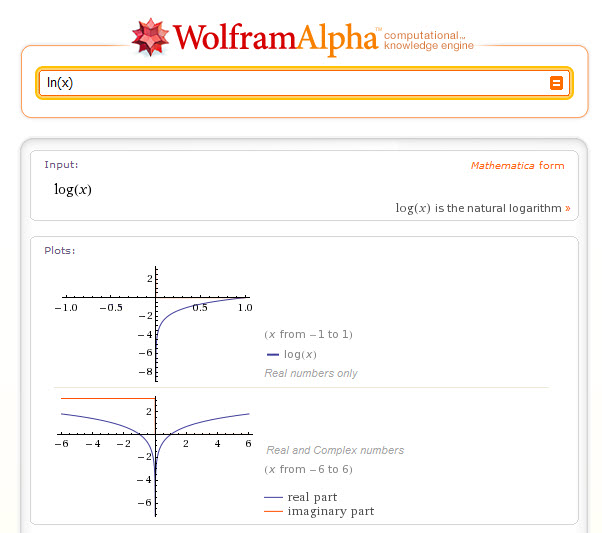At the Wolfram Alpha Workshop at ICTCM, there was universal disappointment about the fact that you cannot get a graph of a logarithm that is only over the real numbers.  We tried everything we could think of to remove the complex part of the graph.

Personally, I have tried and tried and tried and tried to explain the problem with this in the feedback window for Wolfram Alpha, but been universally unsuccessful.   Every time I suggest a change, I am told that the “After review, our internal development group believes the plots for input “log(x)” are correct.” … yes, I know that … that doesn’t mean it’s the answer that most people will be looking for.

I find it ironic that “inverse of e^x” produces the graph we’d like to see, and even gives log(x) as an equivalent.But then ask for a graph of  log(x) or ln(x) and the graph will always include the solution over the complex numbers.What’s worse is that W|A inconsistently decides when to use reals only and when to use both complex and real numbers.  For example, the output for y=ln(x), y=x includes the complex numbered plot, while the output for y=ln(x), y=2x-3 includes only the Reals.  What!?!  Actually, I have some idea why this is … it seems that in some cases, if the extra graph intersects the real part of the log graph, then you get reals only.  If the graphs do not intersect, then you get real+complex.  For example compare the output for y=ln(x), y=2x-3 to the output for y=ln(x), y=2x+5.  On the other hand, when I tried to show a graph transformation, like y=ln(x) with y=ln(x)+4 (including the extra graph y=4x-3), I was back to getting the graphs with complex numbers again. Maddening.We spend a LOT of time in the algebra and precalculus levels working with  transformations of graphs, understanding inverse functions, and specifics like the domain of a graph.  We can’t use Wolfram Alpha for any of these topics with regards to logarithms because of the way the graphs look.  I can live with the fact that W|A uses log(x) instead of ln(x) … it’s not great, and is confusing to students, but I can explain it and live with it.  But as long as the Wolfram Alpha graph includes the complex number system with no way to see the graphs on only the reals, we’ll have to pull out that old-fashioned graphing calculator to teach this section, and that’s a shame.

I’ve also heard the argument that we should just include the domain we want to see in the W|A input.  For example, y=ln(x), x>0.  But how is a student, learning logs for the first time, supposed to recognize that this is required?  After all, the graph they see when they first try W|A with y=ln(x) leads them to believe that y=ln(x) has a domain that includes all real numbers but zero.  This argument also means that to show graph transformations, we need to use much more complicated graphing commands, restricting each domain separately (to tell you the truth, I have not yet figured out a way to do it … although I suspect it’s possible).

It seems to me that there are two obvious solutions to this math teaching nightmare, and I can’t imagine why either one wouldn’t serve all parties using Wolfram Alpha (both high-level mathematicians, and the rest of us):

Solution #1: Use a toggle-able option to see the graph with only reals or both complex and reals  (I would prefer a default to the Real numbers graph, since my guess would be that the majority of the world’s population would be looking for that one).Solution #2: Display TWO graphs.  Show a graph of the logarithm that is only on the real number system.  Then, below it, show a graph that includes both the complex and real number systems.That’s all – end of rant.  This is the one thing I absolutely hate about Wolfram Alpha.  And I’m guessing that I’m not alone here.  Please can’t we just find a solution without hearing “After review, our internal development group believes the plots for input “log(x)” are correct.” again?

Possibly Related Posts: